# RD Sharma Class 10 Ex 8.3 Solutions Chapter 8 Quadratic Equations

In this chapter, we provide RD Sharma Class 10 Ex 8.3 Solutions Chapter 8 Quadratic Equations for English medium students, Which will very helpful for every student in their exams. Students can download the latest RD Sharma Class 10 Ex 8.3 Solutions Chapter 8 Quadratic Equations pdf, Now you will get step by step solution to each question.

# Chapter 8: Quadratic Equations Exercise – 8.3

### Question: 1

Find the roots of the equation (x – 4) (x + 2) = 0

### Solution:

The given equation is (x – 4) (x + 2) = 0

Either x – 4 = 0 therefore x = 4

Or, x + 2 = 0, therefore x = – 2

The roots of the above mentioned quadratic equation are 4 and -2 respectively.

### Question: 2

Find the roots of the equation (2x + 3) (3x – 7) = 0

### Solution:

The given equation is (2x + 3) (3x – 7) = 0.

Either 2x + 3 = 0,

Therefore x = – 3/2

Or, 3x -7 = 0, therefore x = 7/3

The roots of the above mentioned quadratic equation are x = -3/2 and x = 7/3 respectively.

### Question: 3

Find the roots of the quadratic equation 3x– 14x – 5 = 0

### Solution:

The given equation is 3x– 14x – 5 = 0

= 3x– 14x – 5 = 0

= 3x– 15x + x – 5 = 0

= 3x(x – 5) + 1(x – 5) = 0 = (3x + 1)(x – 5) = 0

Either 3x + 1 = 0 therefore x = -1/3

Or, x-5 =0 therefore x = 5

The roots of the given quadratic equation are 5 and x = – 1/3 respectively.

### Question: 4

Find the roots of the equation 9x– 3x – 2 = 0.

### Solution:

The given equation is 9x– 3x – 2 = 0.

= 9x– 3x – 2 = 0.

= 9x2 – 6x + 3x – 2 = 0

= 3x (3x – 2) + 1(3x – 2) = 0

= (3x – 2)(3x + 1) = 0

Either, 3x – 2 = 0 therefore x = 2/3

Or, 3x + 1= 0  therefore x = -1/3

The roots of the above mentioned quadratic equation are x = 2/3 and x = -1/3 respectively.

### Question: 5

Find the roots of the quadratic equation

### Solution:

The given equation is

Cancelling out the like terms on both the sides of the numerator. We get,

= x2+ 4x – 5 = 7

= x2+ 4x – 12 = 0

= x+ 6x – 2x – 12 = 0

= x(x + 6) – 2(x – 6) = 0

= (x + 6)(x – 2) = 0

Either x + 6 = 0

Therefore x = -6 Or, x – 2 = 0

Therefore x = 2

The roots of the above mentioned quadratic equation are 2 and – 6 respectively

### Question: 6

Find the roots of the equation 6x+ 11x + 3 = 0.

### Solution:

The given equation is 6x+ 11x + 3 = 0.

= 6x+ 11x + 3 = 0.

= 6x+ 9x + 2x + 3 = 0

= 3x (2x + 3) + 1(2x + 3) = 0

= (2x +3) (3x + 1) = 0

Either, 2x + 3 = 0

Therefore x = -3/2

Or, 3x + 1= 0 therefore x = -1/3

The roots of the above mentioned quadratic equation are x = -3/2 and x = -1 /3 respectively.

### Question: 7

Find the roots of the equation 5x– 3x – 2 = 0

### Solution:

The given equation is 5x– 3x – 2 = 0.

= 5x– 3x – 2 = 0.

= 5x2 – 5x + 2x – 2 = 0

= 5x(x – 1) + 2(x – 1) = 0

= (5x + 2)(x – 1) = 0

Either 5x + 2 = 0 therefore

x = -2/5 Or, x -1= 0

Therefore x = 1

The roots of the above mentioned quadratic equation are 1 and x = -2/5 respectively.

### Question: 8

Find the roots of the equation 48x– 13x – 1 = 0

### Solution:

The given equation is 48x– 13x – 1 = 0.

= 48x– 13x – 1 = 0.

= 48x– 16x + 3x – 1 = 0.

= 16x(3x – 1) + 1(3x – 1) = 0

= (16x + 1)(3x – 1) = 0

Either 16x + 1 = 0 therefore x = -1/16

Or, 3x-1=0 Therefore x = 1/3

The roots of the above mentioned quadratic equation are x = -1/16 and x = 1/3 respectively.

### Question: 9

Find the roots of the equation 3x= -11x – 10

### Solution:

The given equation is 3x= -11x – 10 = 3x2

= -11x – 10 = 3x+ 11x + 10 = 0

= 3x+ 6x + 5x + 10 = 0

= 3x(x + 2) + 5(x + 2) = 0

= (3x + 2)(x + 2) = 0

Either 3x + 2 = 0 therefore x = -2/3

Or, x + 2 = 0 therefore x = -2

The roots of the above mentioned quadratic equation are x = -2/3 and -2 respectively.

### Question: 10

Find the roots of the equation 25x(x + 1) = – 4

### Solution:

The given equation is 25x(x + 1) = 4

= 25x(x + 1) = -4

= 25x+ 25x + 4 = 0

= 25x+ 20x + 5x + 4 = 0

= 5x (5x + 4) + 1(5x + 4) = 0

= (5x + 4)(5x + 1) = 0

Either 5x + 4 = 0 therefore x = – 4/5

Or, 5x + 1 = 0 therefore x = -1 /5

The roots of the quadratic equation are x = – 4/5 and x = (-1)/5 respectively.

### Question: 11

Find the roots of the quadratic equation### Solution:

The given equation is

Cross multiplying both the sides.

We get,

= 2 = 3x(x – 2)

= 2 = 3x– 6x

= 3x2– 6x – 2 = 0

= 3x2– 3x – 3x – 2 = 0

The roots of the above mentioned quadratic equation are### Question: 12

Find the roots of the quadratic equation### Solution:

The given equation is

The roots of the above mentioned quadratic equation are### Question: 13

Find the roots of the quadratic equation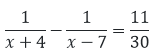### Solution:

The given equation is

Cancelling out the like numbers on both the sides of the equation

= x– 3x – 28 = – 30

= x– 3x – 2 = 0

= x– 2x – x – 2 = 0

= x(x – 2) – 1(x – 2) = 0

= (x – 2)(x – 1) = 0

Either x – 2 = 0

Therefore x = 2 Or, x-1 = 0

Therefore x = 1

The roots of the above mentioned quadratic equation are 1 and 2 respectively.

### Question: 14

Find the roots of the quadratic equation a2x– 3abx + 2b= 0

### Solution:

The given equation is a2x– 3abx + 2b= 0

= a2x– 3abx + 2b= 0

= a2x– abx – 2abx + 2b= 0

= ax(ax – b) – 2b(ax – b) = 0

= (ax – b)(ax – 2b) = 0

Either ax – b = 0

Therefore x = b/a Or, ax – 2b = 0

Therefore x = 2/ba

The roots of the quadratic equation are x = 2/ba and x = b/a respectively.

### Question: 15

Find the roots of the 4x+ 4bx – (a– b2) = 0

### Solution:

= 4(a– b2) = -4(a – b)(a + b) = -2(a-b)*2(a + b)

=2(b – a)*2(b + a) = 4x2+ (2(b – a) + 2(b + a)) – (a – b)(a + b) = 0

= 4x2  + 2(b – a)x++ 2(b + a)x + (b – a)(a + b) = 0

= 2x(2x + (b – a)) +(a + b)(2x + (b – a)) = 0

= (2x + (b – a))(2x + b + a) = 0

Either, (2x + (b – a)) = 0

Therefore x = (a – b)/2 Or, (2x + b + a) = 0

Therefore x = (- a – b)/2

The roots of the above mentioned quadratic equation are x = (- a – b)/2 and x = (a – b)/2 respectively.

### Question: 16

Find the roots of the equation ax+ (4a– 3b)x – 12ab = 0

### Solution:

The given equation is ax+ (4a– 3b)x – 12ab = 0

= ax+ (4a– 3b)x – 12ab = 0

= ax+ 4a2x – 3bx – 12ab = 0

= ax(x – 4a) – 3b(x – 4a) = 0

= (x – 4a)(ax – 4b) = 0

Either x – 4a = 0

Therefore x = 4/a Or, ax – 4b = 0

Therefore x = 4b/a

The roots of the above mentioned quadratic equation are x = 4b/a and 4a respectively.

### Question: 17

Find the roots of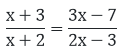### Solution:

The given equation is= (x + 3)(2x – 3) = (x + 2)(3x – 7)

= 2x– 3x + 6x – 9 = 3x– x – 14

= 2x+ 3x – 9 = 3x– x – 14

= x– 3x – x – 14 + 9 = 0

= x– 5x + x – 5 = 0

= x(x – 5) + 1(x – 5) = 0

= (x – 5)(x + l) – 0

Either x – 5 – 0 or x + 1 = 0 x = 5 and x = -1

The roots of the above mentioned quadratic equation are 5 and -1 respectively.

### Question: 18

Find the roots of the equation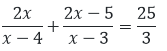### Solution:

The given equation is

= 3(4x– 19x + 20) = 25(x– 7x + 12)

= 12x– 57x + 60 = 25x2 – 175x + 300

= 13x– 78x – 40x + 240 = 0

= 13x– 118x + 240 = 0

= 13x– 78x – 40x + 240 = 0

= 13x(x – 6) – 40(x – 6) = 0

= (x – 6)(13x – 40) = 0

Either x – 6 = 0

Therefore x = 6 Or, 13x – 40 = 0

Therefore x = 40/13

The roots of the above mentioned quadratic equation are 6 and 40/13 respectively.

### Question: 19

Find the roots of the quadratic equation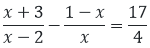### Solution:

The given equation is

= 4(2x+ 2) = 17(x– 2x)

= 8x+ 8 = 17x– 34x

= 9x– 34x – 8 = 0

= 9x– 36x + 2x – 8 = 0

= 9x(x – 4) + 2(x – 4) = 0

= (9x + 2)(x – 4) = 0

Either 9x + 2 = 0

Therefore x = (-2)/9 Or, x – 4 = 0

Therefore x = 4

The roots of the above mentioned quadratic equation are x = (- 2)/9 and 4 respectively.

### Question: 20

Find the roots of the quadratic equation### Solution:

The equation is

= x(3x – 5) = 6(x– 3x + 2)

= 3x– 5x = 6x– 18x + 12

= 3x– 13x + 12 = 0

= 3x– 9x – 4x + 12 = 0

= 3x(x – 3) – 4(x – 3) = 0

= (x – 3)(3x – 4) = 0

Either x – 3 = 0

Therefore x = 3 Or, 3x – 4 = 0

Therefore 4/3. The roots of the above mentioned quadratic equation are 3 and 4/3 respectively.

### Question: 21

Find the roots of the quadratic equation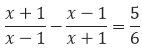### Solution:

The equation is

= 6(4x) = 5(x– 1) = 24x

= 5x– 5 = 5x– 24x – 5 =0

= 5x– 25x + x – 5 = 0

= 5x(x – 5) + 1(x – 5) = 0

= (5x + 1)(x – 5) = 0

Either x – 5 = 0

Therefore x = 5 Or, 5x + 1 = 0

Therefore x = −1/5

The roots of the above mentioned quadratic equation are x = −1/5 and 5 respectively.

### Question: 22

Find the roots of the quadratic equation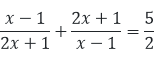### Solution:

The equation is

= 2(5x+ 2x + 2) = 5(2x– x – 1)

= 10x+ 4x + 4 = 10x– 5x – 5

Cancelling out the equal terms on both sides of the equation.

We get, = 4x + 5x + 4 + 5 = 0

= 9x + 9 = 0

= 9x = -9 X = -1 X = -1 is the only root of the given equation.

### Question: 23

Find the roots of the quadratic equation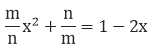### Solution:

The given equation is

= m2x+ 2mnx + (n– mn) = 0

Now we solve the above quadratic equation using factorization method

Therefore

Now, one of the products must be equal to zero for the whole product to be zero for the whole product to be zero. Hence, we equate both the products to zero in order to find the value of x.

The roots of the above mentioned quadratic equation are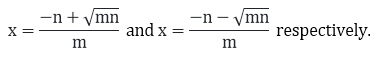### Question: 24

Find the roots of the quadratic equation### Solution:

The given equation is

= (2x– 2x(a + b) + a+ b2)ab

= (a+ b2)(x– (a + b)x + ab)

= (2abx– 2abx(a + b) + ab(a+ b2))

= (a+ b2)(x– (a+ b2)(a + b)x + (a+ b2)(ab)

= (a+ b– 2ab)x – (a + b)(a+ b– 2ab)x = 0

= (a – b)2x– (a + b)(a + b)2x= 0

= x(a – b)2(x – (a + b)) = 0

= x(x – (a + b)) = 0

Either x = 0 Or, (x – (a + b)) = 0

Therefore x = a + b

The roots of the above mentioned quadratic equation are 0 and a + b respectively.

### Question: 25

Find the roots of the quadratic equation

### Solution:

The given equation is

Cancelling out the like terms on both the sides of numerator and denominator.

We get,

= (x – 1)(x – 4) = 18

= x– 4x – x + 4 = 18

= x– 5x – 14 = 0

= x– 7x + 2x – 14 = 0

= x(x – 7) + 2(x – 7) = 0

= (x – 7)(x + 2) = 0

Either x – 7 = 0

Therefore x = 7 Or, x + 2 = 0

Therefore x = -2

The roots of the above mentioned quadratic equation are 7 and -2 respectively.

### Question: 26

Find the roots of the quadratic equation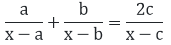### Solution:

The given equation is

= (x – c)(ax – 2ab + bx)

= 2c(x– bx – ax + ab)

= (a + b)x– 2abx – (a + b)cx + 2abc

= 2cx– 2c(a + b)x + 2abc

### Question: 27

Find the roots of the Question x+ 2ab = (2a + b)x

### Solution:

The given equation is x+ 2ab = (2a + b)x

= x+ 2ab = (2a+b)x

= x– (2a + b)x + 2ab = 0

= x– 2ax – bx + 2ab = 0

= x(x – 2a) – b(x – 2a) = 0

= (x – 2a)(x – b) = 0

Either x – 2a = 0

Therefore x = 2a Or, x – b = 0

Therefore x = b

The roots of the above mentioned quadratic equation are 2a and b respectively.

### Question: 28

Find the roots of the quadratic equation (a + b)2x– 4abx – (a – b)2  = 0

### Solution:

The given equation is (a + b)2x– 4abx – (a – b)2  = 0

= (a + b)2x– 4abx – (a – b)2  = 0

= (a + b)2x– ((a + b)– (a – b)2)x – (a – b)= 0

= (a + b)2x– (a + b)x + (a – b)x – (a – b)= 0

= (a + b)2x(x – 1) (a + b)2 (x – 1) = 0

= (x – 1) (a + b)2x +( a + b)2) = 0

Either x – 1 = 0

Therefore x = 1 Or, (a + b)2x + (a + b)2) = 0

The roots of the above mentioned quadratic equation are –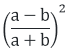and 1 respectively.

### Question: 29

Find the roots of the quadratic equation a(x+ 1) – x(a+ 1) = 0

### Solution:

The given equation is a(x+ 1) – x(a+ 1) = 0

= ax+ a – a2x – x = 0

= ax(x – a) – 1(x – a) = 0

= (x – a)(ax – 1) = 0

Either x – a = 0

Therefore x = a Or, ax – 1 = 0

Therefore x = 1/a

The roots of the above mentioned quadratic equation are  (a) and x = 1/a respectively.

### Question: 30

Find the roots of the quadratic equation

### Solution:

The given equation is

Either x + a = 0

Therefore x = -a Or, (x + 1/a) = 0

Therefore x = 1/a. The roots of the above mentioned quadratic equation are x = 1/a and – a respectively.

### Question: 31

Find the roots of the quadratic equation abx+ (b– ac)x – bc = 0

### Solution:

The given equation is abx+ (b– ac)x – bc = 0

= abx+ b2x – acx – bc = 0

= bx (ax + b) – c (ax + b) = 0

= (ax + b)(bx – c) = 0

Either, ax + b = 0

Therefore x = −b/a Or, bx – c = 0

Therefore x = c/b

The roots of the above mentioned quadratic equation are x = c/b and x = −b/a respectively.

### Question: 32

Find the roots of the quadratic equation a2b2x+ b2x – a2x – 1 = 0

### Solution:

The given equation is a2b2x+ b2x – a2x – 1 = 0

= b2x(a2x + 1) -1 (a2x + 1) = (a2x+1)( b2x-1) =0

Either (a2x + 1) = 0

Therefore x = -1/a2Or, ( b2x-1) = 0

Therefore x = -1/b2

The roots of the above mentioned quadratic equation are x = 1/b2 and x = (-1)/a2respectively.

All Chapter RD Sharma Solutions For Class10 Maths

I think you got complete solutions for this chapter. If You have any queries regarding this chapter, please comment on the below section our subject teacher will answer you. We tried our best to give complete solutions so you got good